# CAA_OUTPUT

Specifies output parameters for Computational Aero-Acoustics (CAA) post-processing.

AcuSolve Command

## Syntax

CAA_OUTPUT("name") {parameters...}

User-given name.

## Parameters

coordinates or coord (array) [no default]
An array of sample points. Each row contains a unique identification number and the x, y, and z coordinates of a point in the global xyz coordinate system.
directions or dir (array) [no default]
An array of outward normal direction vectors corresponding to the coordinates array. Each row contains an identification number number and the x, y, and z components of a vector in the global xyz coordinate system. Required by some of the output quantities.
gap (real) >=0 [=0]
Tolerance for sample points falling outside of all elements.
output_coordinates (boolean) [=off]
Flag specifying whether to output the coordinates.
output_velocity (boolean) [=off]
Flag specifying whether to output the velocity.
output_pressure (boolean) [=off]
Flag specifying whether to output the pressure.
output_momentum_stress (boolean) [=off]
Flag specifying whether to output the momentum stress.
output_total_stress (boolean) [=off]
Flag specifying whether to output the total stress.
output_lighthill_stress (boolean) [=off]
Flag specifying whether to output the Lighthill stress.
output_reduced_lighthill_stress (boolean) [=off]
Flag specifying whether to output the reduced Lighthill stress.
output_div_momentum_stress (boolean) [=off]
Flag specifying whether to output the divergence of the momentum stress.
output_div_total_stress (boolean) [=off]
Flag specifying whether to output the divergence of the total stress.
output_div_lighthill_stress (boolean) [=off]
Flag specifying whether to output the divergence of the Lighthill stress.
output_div_reduced_lighthill_stress (boolean) [=off]
Flag specifying whether to output the divergence of the reduced Lighthill stress.
output_normal_momentum_flux (boolean) [=off]
Flag specifying whether to output the normal component of the momentum flux.
output_normal_momentum_flux_rate (boolean) [=off]
Flag specifying whether to output the normal component of the momentum flux rate.
output_normal_div_total_stress (boolean) [=off]
Flag specifying whether to output the normal component of the divergence of the total stress.
output_fwh_monopole (boolean) [=off]
Flag specifying whether to output the monopole term of the Ffowcs Williams Hawkings acoustics equations.
output_fwh_dipole (boolean) [=off]
Flag specifying whether to output the dipole term of the Ffowcs Williams Hawkings acoustics equations.
output_ei_na (boolean) [=off]
Flag specifying whether to output the element-integrated volume.
output_ei_na_velocity (boolean) [=off]
Flag specifying whether to output the element-integrated velocity.
output_ei_na_pressure (boolean) [=off]
Flag specifying whether to output the element-integrated pressure.
output_ei_na_momentum_stress (boolean) [=off]
Flag specifying whether to output the element-integrated momentum stress.
output_ei_na_total_stress (boolean) [=off]
Flag specifying whether to output the element-integrated total stress.
output_ei_na_lighthill_stress (boolean) [=off]
Flag specifying whether to output the element-integrated Lighthill stress.
output_ei_na_reduced_lighthill_stress (boolean) [=off]
Flag specifying whether to output the element-integrated reduced Lighthill stress.
output_ei_na_div_momentum_stress (boolean) [=off]
Flag specifying whether to output the element-integrated divergence of the momentum stress.
output_ei_na_div_total_stress (boolean) [=off]
Flag specifying whether to output the element-integrated divergence of the total stress.
output_ei_na_div_lighthill_stress (boolean) [=off]
Flag specifying whether to output the element-integrated divergence of the Lighthill stress.
output_ei_na_div_reduced_lighthill_stress (boolean) [=off]
Flag specifying whether to output the element-integrated divergence of the reduced Lighthill stress.
output_ei_gna_div_momentum_stress (boolean) [=off]
Flag specifying whether to output the element-integrated shape function gradient contracted with the divergence of the momentum stress.
output_ei_gna_div_total_stress (boolean) [=off]
Flag specifying whether to output the element-integrated shape function gradient contracted with the divergence of the total stress.
output_ei_gna_div_lighthill_stress (boolean) [=off]
Flag specifying whether to output the element-integrated shape function gradient contracted with the divergence of the Lighthill stress.
output_ei_gna_div_reduced_lighthill_stress (boolean) [=off]
Flag specifying whether to output the element-integrated shape function gradient contracted with the divergence of the reduced Lighthill stress.
output_si_na (boolean) [=off]
Flag specifying whether to output the surface-integrated area.
output_si_na_normal (boolean) [=off]
Flag specifying whether to output the surface-integrated normal.
output_si_na_pressure (boolean) [=off]
Flag specifying whether to output the surface-integrated pressure.
output_si_na_normal_pressure (boolean) [=off]
Flag specifying whether to output the surface-integrated normal-pressure.
output_si_na_normal_momentum_flux (boolean) [=off]
Flag specifying whether to output the surface-integrated normal component of the momentum flux.
output_si_na_normal_momentum_flux_rate (boolean) [=off]
Flag specifying whether to output the surface-integrated normal component of the momentum flux rate.
output_si_na_normal_div_total_stress (boolean) [=off]
Flag specifying whether to output the surface-integrated normal component of the divergence of the total stress.
output_si_na_fwh_monopole (boolean) [=off]
Flag specifying whether to output the surface-integrated monopole term of the Ffowcs Williams Hawkings acoustics equations.
output_si_na_fwh_dipole (boolean) [=off]
Flag specifying whether to output the surface-integrated dipole term of the Ffowcs Williams Hawkings acoustics equations.
reference_density or ref_dens (real) [=1.225]
Reference density. Required by some of the output quantities.
reference_pressure or ref_pres (real) [=0]
Reference pressure. Required by some of the output quantities.
reference_sound_speed or sound (real) [=330]
Reference sound speed. Required by some of the output quantities.
output_frequency or out_freq (integer) >=0 [=1000]
Time step frequency at which to output CAA data. If zero, this option is ignored.
output_time_interval or out_intv (real) >=0 [=0]
Time frequency at which to output CAA data. If zero, this option is ignored.
num_saved_states or save (integer) >=0 [=0]
Number of CAA data files to keep. If zero, all are kept.

## Description

This command specifies output data to support Computational Aero-Acoustics (CAA) post-processing. For example,
CAA_OUTPUT( "sample points" ) {
coordinates                                = { 1, 0., 0., 0. ;
2, 1., 0., 0. ;
11, 0., 1., 0. ;
12, 1., 1., 0. ; }
directions                                 = { 1, 0., 0., 1. ;
2, 0., 0., 1. ;
11, 0., 0., 1. ;
12, 0., 0., 1. ; }
gap                                        = 0
output_coordinates                         = on
output_velocity                            = on
output_pressure                            = off
output_momentum_stress                     = off
output_total_stress                        = off
output_lighthill_stress                    = off
output_reduced_lighthill_stress            = off
output_div_momentum_stress                 = off
output_div_total_stress                    = off
output_div_lighthill_stress                = off
output_div_reduced_lighthill_stress        = off
output_normal_momentum_flux                = off
output_normal_momentum_flux_rate           = off
output_normal_div_total_stress             = off
output_fwh_monopole                        = off
output_fwh_dipole                          = off
output_ei_na                               = off
output_ei_na_velocity                      = off
output_ei_na_pressure                      = off
output_ei_na_momentum_stress               = off
output_ei_na_total_stress                  = off
output_ei_na_lighthill_stress              = off
output_ei_na_reduced_lighthill_stress      = off
output_ei_na_div_momentum_stress           = off
output_ei_na_div_total_stress              = off
output_ei_na_div_lighthill_stress          = off
output_ei_na_div_reduced_lighthill_stress  = off
output_ei_gna_div_momentum_stress          = off
output_ei_gna_div_total_stress             = off
output_ei_gna_div_lighthill_stress         = off
output_ei_gna_div_reduced_lighthill_stress = off
output_si_na                               = off
output_si_na_normal                        = off
output_si_na_normal_pressure               = off
output_si_na_normal_momentum_flux          = off
output_si_na_normal_momentum_flux_rate     = off
output_si_na_normal_div_total_stress       = off
output_si_na_fwh_monopole                  = off
output_si_na_fwh_dipole                    = off
reference_density                          = 1.225
reference_pressure                         = 0
reference_sound_speed                      = 330
output_frequency                           = 1
output_time_interval                       = 0
num_saved_states                           = 0
}

A set of sample points is specified by the coordinates parameter, which is a four-column array corresponding to an identification number and the x, y, and z coordinates of each sample point, one row per point. The identification number may be any arbitrary but unique (within the set) integer. Negative numbers are acceptable. The coordinates are in the global xyz coordinate system. This is the same format as the coordinates parameter of the COORDINATE command, but the sample points do not have to be a subset of the nodal coordinates nor related to them in any other way. The coordinates parameter is mandatory. Within AcuPrep, an element is found for each sample point and interpolation shape functions are computed. If a sample point falls outside all fluid elements, then the closest element face is found, up to a distance specified by gap. An error is issued if no element is found.

Some of the output fields require a direction vector. If needed, the directions parameter must be given as a four column array: identification number, and the x, y, and z components of the direction. The identification numbers must be the same set as given by coordinates. The parameters reference_density, reference_pressure and reference_sound_speed provide the required data for certain fields. Any of these extra parameters that a particular field requires are noted in the table below.

The sample points and normal directions may be read from files. For the above example, the sample points may be placed in a file, such as channel.caa.crd:
1 0. 0. 0.
2 1. 0. 0.
11 0. 1. 0.
12 1. 1. 0.
and the normal directions placed in another file, such as channel.caa.dir:
1 0. 0. 1.
2 0. 0. 1.
11 0. 0. 1.
12 0. 0. 1.
Both may then by read by:
CAA_OUTPUT( "sample points" ) {
...
}

Since the volume of data written on disk by CAA_OUTPUT could be very large, this command provides the option of selecting which data are to be written on disk via the parameters output_coordinates through output_si_na_fwh_dipole. The default is to not output any fields. The output quantities and their required data are defined in the following table:

Name DOFs Definition Data
coordinates 3 x
velocity 3 u
pressure 1 p
momentum_stress 6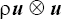total_stress 6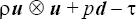lighthill_stress 6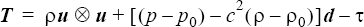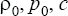reduced_lighthill_stress 6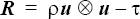div_momentum_stress 3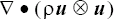div_total_stress 3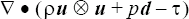div_lighthill_stress 3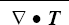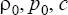div_reduced_lighthill_stress 3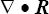normal_momentum_flux 1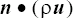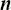normal_momentum_flux_rate 1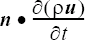normal_div_total_stress 1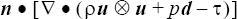fwh_monopole 1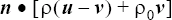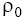fwh_dipole 1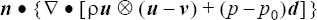ei_na 1ei_na_velocity 3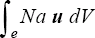ei_na_pressure 1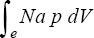ei_na_momentum_stress 6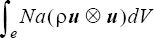ei_na_total_stress 6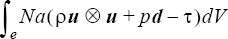ei_na_lighthill_stress 6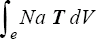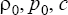ei_na_reduced_lighthill_stress 6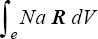ei_na_div_momentum_stress 3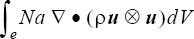ei_na_div_total_stress 3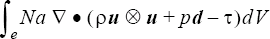ei_na_div_lighthill_stress 3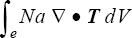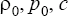ei_na_div_reduced_lighthill_stress 3ei_gna_div_momentum_stress 1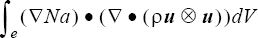ei_gna_div_total_stress 1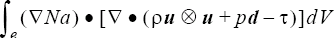ei_gna_div_lighthill_stress 1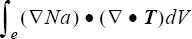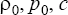ei_gna_div_reduced_lighthill_stress 1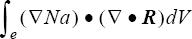si_na 1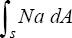si_na_normal 3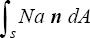si_na_pressure 1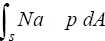si_na_normal_pressure 3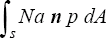si_na_normal_momentum_flux 1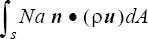si_na_normal_momentum_flux_rate 1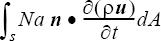si_na_normal_div_total_stress 1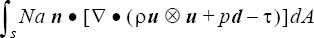si_na_fwh_monopole 1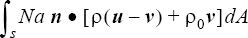si_na_fwh_dipole 1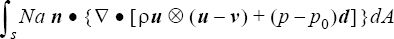where x is the coordinate vector; u is the velocity; v is the mesh velocity; n is the outward normal direction, given by directions; p is the pressure;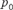is the reference pressure, given by reference_pressure; ρ is the density;is the reference density, given by reference_density; c is the reference speed of sound, given by reference_sound_speed; d is the Kronecker delta (identity matrix);is the viscous stress tensor (including turbulence effects); and Na is the element shape function. The stress-type output quantities with 6 DOFs are ordered as xx, yy, zz, xy, yz, zx. Due to the mesh velocity term the fwh_monopole variables do not contain the usual time derivative.

The variables starting with "ei_" are element-integrated. The elements that contribute to the integration are specified by the CAA_ELEMENT_OUTPUT commands that reference the qualifier of the CAA_OUTPUT command.

The element connectivity is defined in terms of the sample points in coordinates. If any of these points is not referenced by the element connectivity then the element integrals are zero at that point. Similarly, variables starting with "si_" are surface-integrated, and the surfaces that contribute to the integration are specified by the CAA_SURFACE_OUTPUT commands that reference the qualifier of the CAA_OUTPUT command. Unreferenced points again yield zero integrals. In both cases, the integrals appearing in the definitions above include sums over the appropriate shape functions. Because of the inclusion of the shape function each integral is local to the sample points and effectively yields a smoothing of the integrand. For example,
CAA_OUTPUT( "sample points" ) {
...
output_ei_na                  = on
output_ei_na_lighthill_stress = on
output_si_na                  = on
output_si_na_fwh_monopole     = on
...
}
CAA_ELEMENT_OUTPUT( "sample elements" ) {
caa_output                    = "sample points"
shape                         = eight_node_brick
}
CAA_SURFACE_OUTPUT( "sample surfaces" ) {
caa_output                    = "sample points"
}